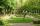# The field

The player crossed the field diagonally and walked the length of 250 m. Calculate the length of the field, circumference if one side of field 25 meters.

a =  248.7 m
o =  547.5 m
S =  6218.7 m2

### Step-by-step explanation:Did you find an error or inaccuracy? Feel free to write us. Thank you!Tips to related online calculators
Pythagorean theorem is the base for the right triangle calculator.

#### You need to know the following knowledge to solve this word math problem:

We encourage you to watch this tutorial video on this math problem:

## Related math problems and questions:

• Rectangular fieldA rectangular field has a diagonal of length 169m. If the length and width are in the ratio 12:5. Find the dimensions of the field, the perimeter of the field and the area of the field.
• RectangleThe length of the rectangle are in the ratio 5:12 and the circumference is 238 cm. Calculate the length of the diagonal and area of rectangle.
• Land - isosceles trapezoidCalculate the building plot's content and perimeter in the form of an isosceles trapezoid with bases 120m, 95m, and height 50m.
• Side and diagonalFind the circumference and the area of the rectangle if given: side a = 8 cm diagonal u = 10 cm.
• SquareCalculate the area of the square shape of the isosceles triangle with the arms 50m and the base 60m. How many tiles are used to pave the square if the area of one tile is 25 dm2?
• BillboardRectangular billboard is 2.5 m long with a diagonal 2.8 m long. Calculate the perimeter and the content area of the billboard.
• Cube wallThe perimeter of one cube wall is 120 meters. Calculate the surface area and the body diagonal of this cube.
• RT 11Calculate the area of right tirangle if its perimeter is p = 45 m and one cathethus is 20 m long.
• The sidesThe sides of the rectangle are in a ratio of 3: 5 and its circumference measures 72 cm. Calculate: a) the size of both sides of the rectangle b) the area of the rectangle c) the length of the diagonals
• Diamond diagonalsCalculate the diamonds' diagonals lengths if the diamond area is 156 cm square, and the side length is 13 cm.
• Rectangle diagonalsIt is given a rectangle with an area of 24 cm2 a circumference of 20 cm. The length of one side is 2 cm larger than the length of the second side. Calculate the length of the diagonal. Length and width are yet expressed in natural numbers.
• Square diagonalCalculate the length of the square diagonal if the perimeter is 172 cm.
• Central park in cityThe city park has the shape of a rectangle of 180 meters in length and 120 meters in width. People make their walk through the center of the park from one corner to the second. Calculate how many meters this way is shorter than they walked along the path
• The rectangleThe rectangle has a circumference of 32 m. One side is 2 m longer than the other side. Calculate the side lengths of this rectangle.
• Rectangular gardenThe sides of the rectangular garden are in ratio 1: 2. The diagonal has a length of 20 meters. Calculate the area and perimeter of the garden.
• The tractorThe tractor sows an average of 1.5 ha per hour. In how many hours does it sows a rectangular trapezoid field with the bases of 635m and 554m and a longer arm 207m?
• Rectangle diagonalThe rectangle, one side of which is 5 cm long, is divided by a 13 cm diagonal into two triangles. Calculate the area of one of these triangles in cm2.